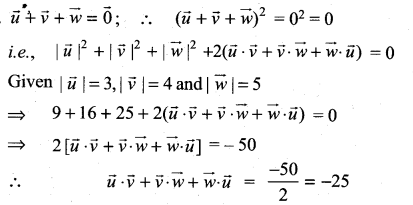# Samacheer Kalvi 11th Maths Solutions Chapter 8 Vector Algebra – I Ex 8.3

## Tamilnadu Samacheer Kalvi 11th Maths Solutions Chapter 8 Vector Algebra – I Ex 8.3

Question 1.
Find $$\vec{a} \cdot \vec{b}$$ when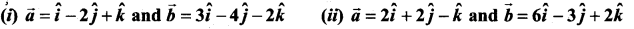Solution: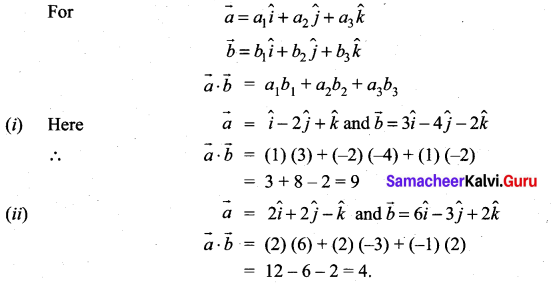Question 2.
Find the value of λ for which the vectors $$\vec{a}$$ and $$\vec{b}$$ are perpendicular, where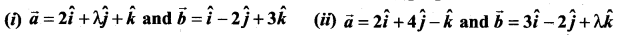Solution:
When $$\vec{a}$$ and $$\vec{b}$$ are ⊥r then $$\vec{a} \cdot \vec{b}$$ = 0
$$\vec{a}$$ ⊥r $$\vec{b}$$ ⇒ $$\vec{a} \cdot \vec{b}$$ = 0
(i) (2) (1) + (λ) (-2) + (1) (3) = 0 ⇒ λ = 5/2
(ii) (2) (3) + (4) (-2) + (-1) (λ) = 0
6 – 8 – λ = 0
-λ – 2 = 0 ⇒ -λ = 2 ⇒ λ = -2

Question 3.
If $$\vec{a}$$ and $$\vec{b}$$ are two vectors such that |$$\vec{a}$$| = 10, |$$\vec{b}$$| = 15 and $$\vec{a} \cdot \vec{b}$$ = 75$$\sqrt{2}$$ , find the angle between $$\vec{a}$$ and $$\vec{a}$$ .
Solution:Question 4.
Find the angle between the vectors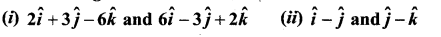Solution: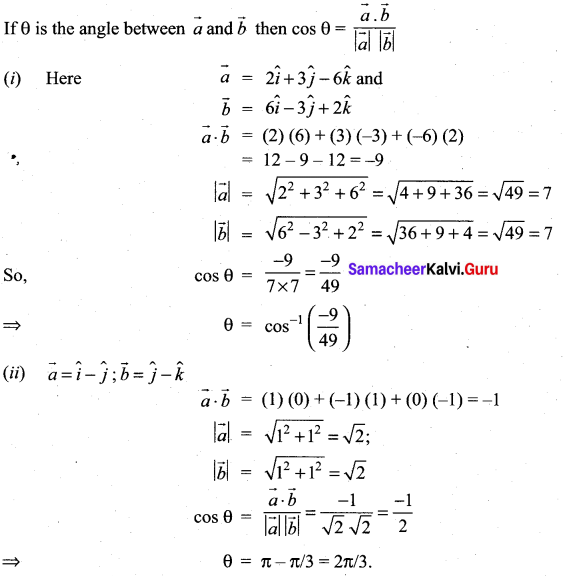Question 5.
If $$\overrightarrow{\boldsymbol{a}}, \overrightarrow{\boldsymbol{b}}, \overrightarrow{\boldsymbol{c}}$$ are three vectors such that $$\vec{a}+2 \vec{b}+\vec{c}=\overrightarrow{0}$$ and $$|\vec{a}|=3,|\vec{b}|=4,|\vec{c}|=7$$ find the angle between $$\vec{a}$$ and $$\vec{b}$$
Solution: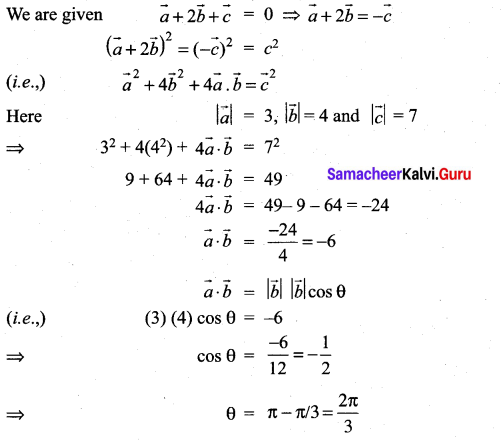Question 6.
Show that the vectors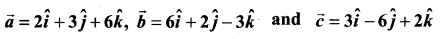are mutually orthogonal.
Solution: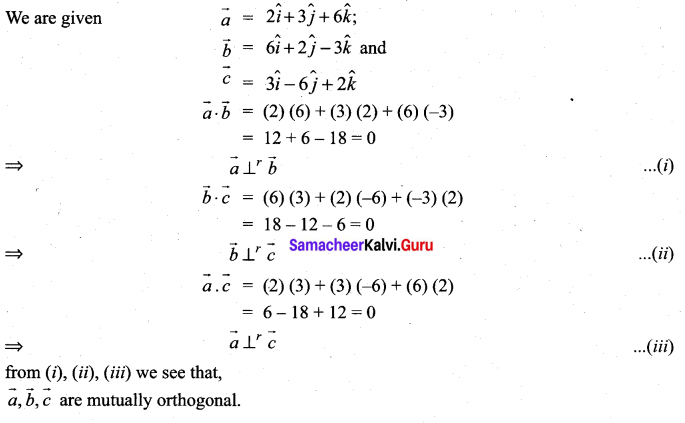Question 7.
Show that the vectors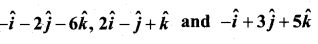form a right angled triangle.
Solution: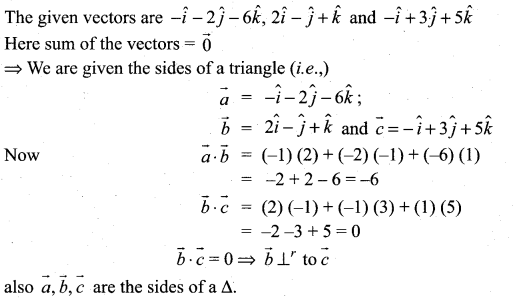So, the given vectors form the sides of a right angled triangle

Question 8.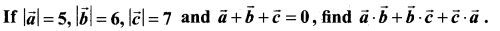Solution: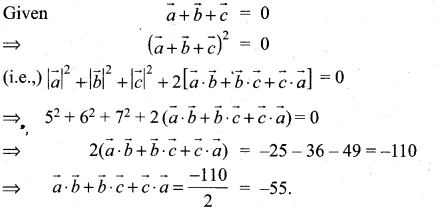Question 9.
Show that the points (2, -1, 3) (4, 3, 1) and (3, 1, 2) are collinear
Solution:
Let the given points be A, B, C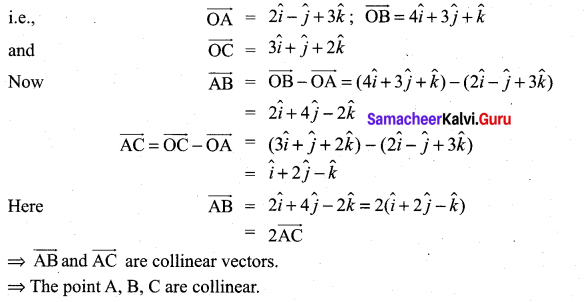Question 10.
If $$\vec{a}, \vec{b}$$ are unit vectors and θ is the angle between them, show that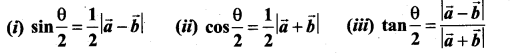Solution: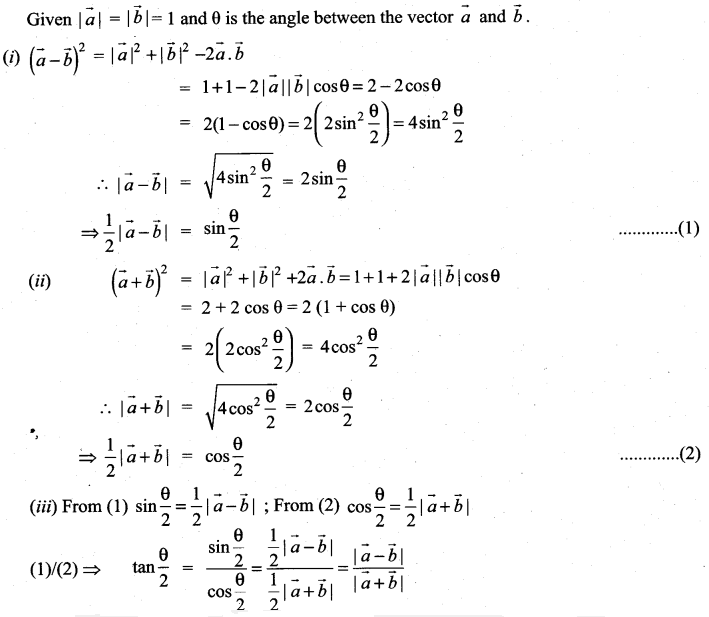Question 11.
Let $$\vec{a}, \vec{b}, \vec{c}$$ be the three vectors such that $$|\vec{a}|=3,|\vec{b}|=4,|\vec{c}|=5$$ and each one of them being perpendicular to the sum of the other two, find $$|\vec{a}+\vec{b}+\vec{c}|$$
Solution: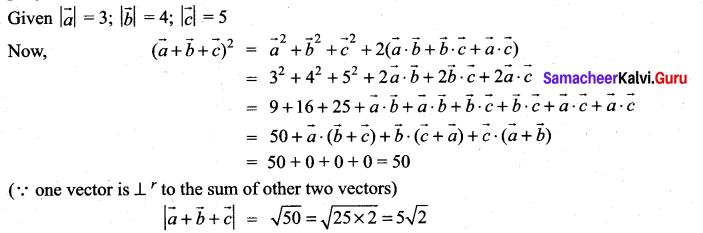Question 12.
Find the projection of the vector $$\hat{i}+3 \hat{j}+7 \hat{k}$$ on the vector $$2 \hat{i}+6 \hat{j}+3 \hat{k}$$
Solution: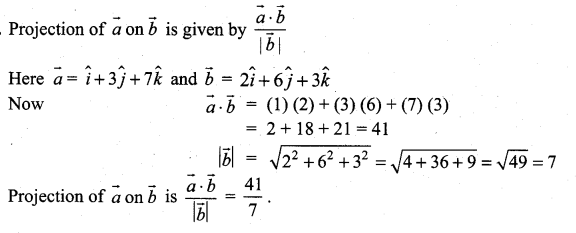Question 13.
Find λ, when the projection of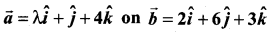is 5 units.
Solution: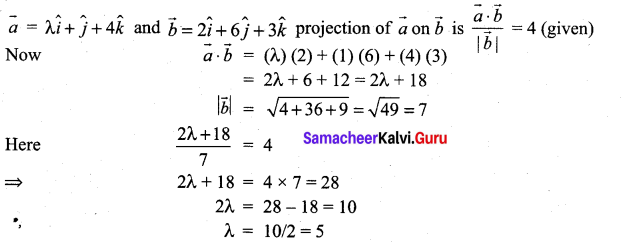Question 14.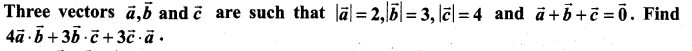Solution: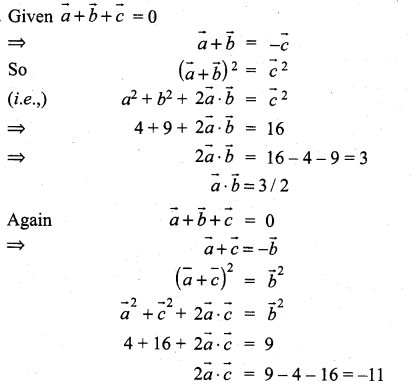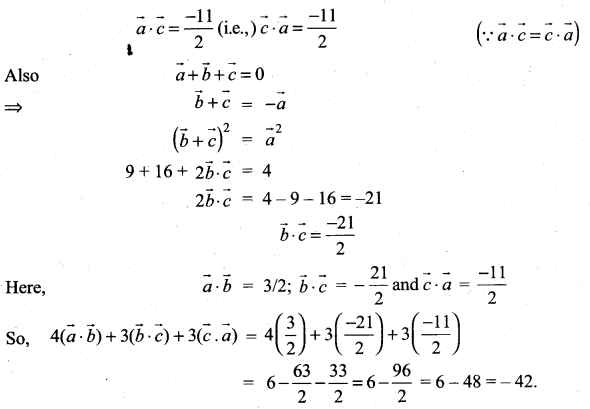### Samacheer Kalvi 11th Maths Solutions Chapter 8 Vector Algebra – I Ex 8.3 Additional Problems

Question 1.
Find λ so that the vectors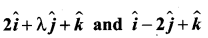are perpendicular to each other.
Solution: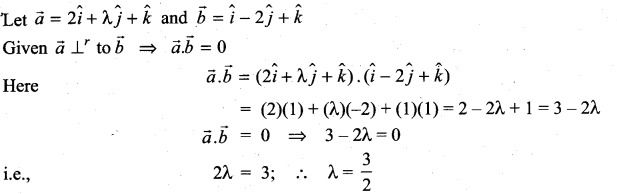Question 2.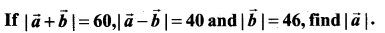Solution: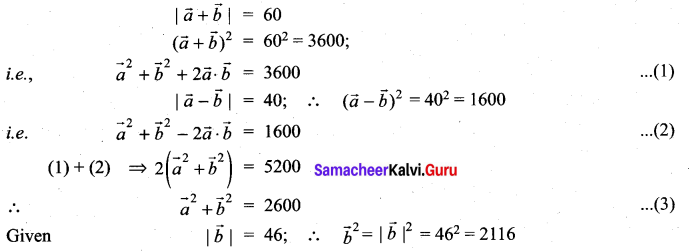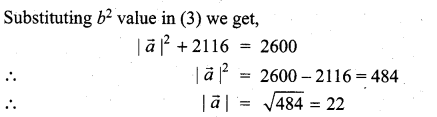Question 3.
If the sum of two unit vectors is a unit vector prove that the magnitude of their difference is $$\sqrt{3}$$
Solution: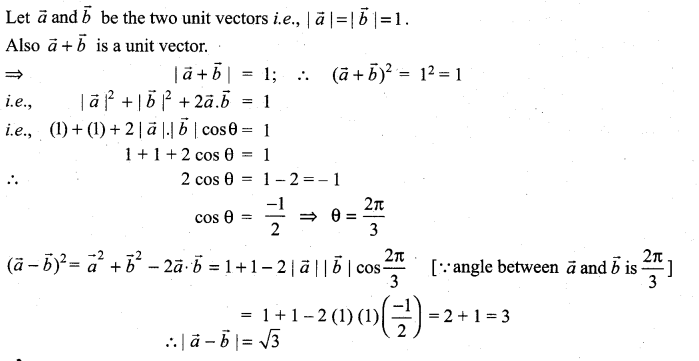Question 4.
Show that the vectors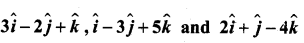form a right angled triangle.
Solution: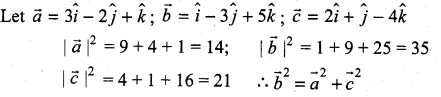⇒ The given vectors form the sides of a right of a right angled triangle.Question 5.
Find the projection of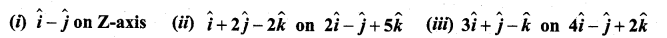Solution: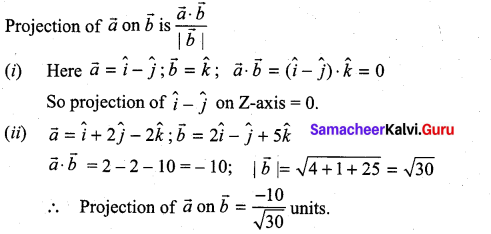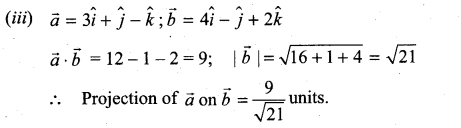Question 6.
Show that the vector $$\hat{i}+\hat{j}+\hat{k}$$ is equally inclined with the coordinate axes.
Solution: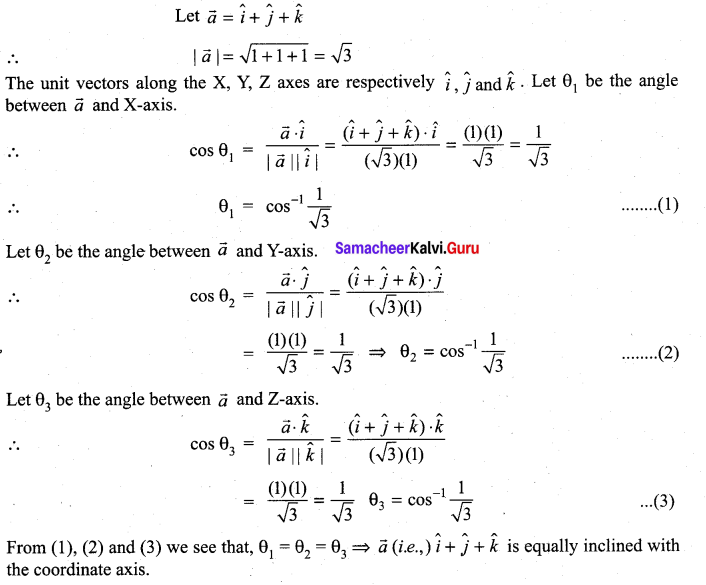Question 7.
If $$\vec{a}, \vec{b}, \vec{c}$$ are three mutually perpendicular unit vectors, then prove that $$|\vec{a}+\vec{b}+\vec{c}|=\sqrt{3}$$
Solution: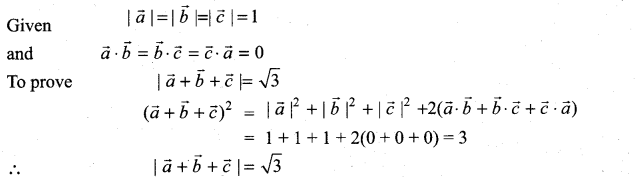Question 8.
Show that the points whose positions vectors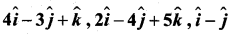from a right angled triangle.
Solution: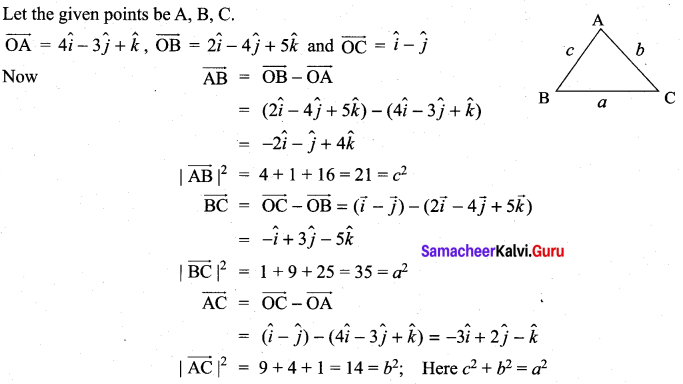⇒ The given points form a right angled triangle.

Question 9.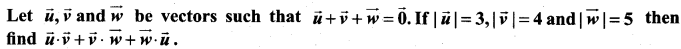Solution: